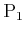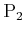## 9.3.3 Randomized Strategies

The fact that some zero-sum games do not have a saddle point is disappointing because regret is unavoidable in these cases. Suppose we slightly change the rules. Assume that the same game is repeatedly played byandover numerous trials. If they use a deterministic strategy, they will choose the same actions every time, resulting in the same costs. They may instead switch between alternative security strategies, which causes fluctuations in the costs. What happens if they each implement a randomized strategy? Using the idea from Section 9.1.3, each strategy is specified as a probability distribution over the actions. In the limit, as the number of times the game is played tends to infinity, an expected cost is obtained. One of the most famous results in game theory is that on the space of randomized strategies, a saddle point always exists for any zero-sum matrix game; however, expected costs must be used. Thus, if randomization is used, there will be no regrets. In an individual trial, regret may be possible; however, as the costs are averaged over all trials, both players will be satisfied.

Subsections
Steven M LaValle 2020-08-14